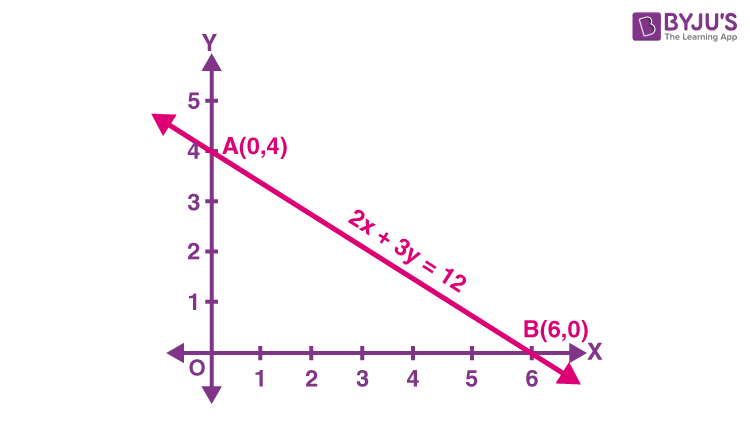# Draw the graph of the equation 2x - 3y = 12. At what point does the graph of the equation cuts the x axis and y axis?

Solution:

Modify the equation as follows:

y = (12 – 2x)/3

For x = 0, y = 4, therefore, (0, 4) lies on the graph. Similarly, for y = 0, x = 6, therefore, (6, 0) lies on the graph.

Now plot the points A (0, 4) and B (6, 0) and join them as shown in the figure below, to get the line AB.From the figure, you can see that the graph (line AB) cuts the x-axis at the point (6, 0) and the y-axis at the point (0, 4).